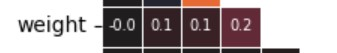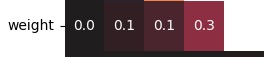# Data Analysis with Python Projects - Medical Data Visualizer - Data Cleaning

Hello!
I’m currently working on Medical Data Visualizer using Vscode, after trying to run the function, there’s some errors in the results :

Diff is 1966 characters long. Set self.maxDiff to None to see it. : Expected different values in heat map.

I’ve tried both filter out (drop) the incorrect data from dataframe, or reverse it by keeping the correct data, but it doesn’t seem to change.

I think the problem is on the data cleaning part, but i’m stuck and can’t for the life of me find where the issues is. I’m new to python so, the solution might be obviousThanks!

``````import pandas as pd
import seaborn as sns
import matplotlib.pyplot as plt
import numpy as np

# Import data

df['overweight'] = df['weight'] / ((df['height'] /100) ** 2 )
df['overweight'] = np.where( df['weight'] / ((df['height'] /100) ** 2 ) > 25, 1, 0)

# Normalize data by making 0 always good and 1 always bad. If the value of 'cholesterol' or 'gluc' is 1, make the value 0. If the value is more than 1, make the value 1.
df['cholesterol'] = np.where( df['cholesterol'] == 1, 0, 1)
df['gluc'] = np.where( df['gluc'] == 1, 0, 1)

# Draw Categorical Plot
def draw_cat_plot():
# Create DataFrame for cat plot using `pd.melt` using just the values from 'cholesterol', 'gluc', 'smoke', 'alco', 'active', and 'overweight'.
df_cat = pd.melt(df, value_vars=['cholesterol','gluc', 'smoke', 'alco', 'active', 'overweight'])

# Group and reformat the data to split it by 'cardio'. Show the counts of each feature. You will have to rename one of the columns for the catplot to work correctly.
df_cat = pd.melt(df, id_vars=['cardio'], value_vars=['cholesterol','gluc', 'smoke', 'alco', 'active', 'overweight'])
df_cat = pd.melt(df, id_vars=['cardio'], value_vars=['cholesterol','gluc', 'smoke', 'alco', 'active', 'overweight']).value_counts().reset_index()
df_cat['value'] = df_cat['value'].astype(str) #To avoid errors on replit, Needs to change to strings, otherwise it'll results on error
df_cat.rename(columns={'count': 'total'}, inplace=True)
df_cat.sort_values(by='variable', inplace=True)

# Draw the catplot with 'sns.catplot()'
cat_plot = sns.catplot( data=df_cat, x='variable', y='total', kind='bar', col='cardio' , hue='value' , palette='Set1' )
cat_plot.set_axis_labels('variable', 'total')

# Get the figure for the output
fig = cat_plot.figure

# Do not modify the next two lines
fig.savefig('catplot.png')

return fig

# Draw Heat Map
def draw_heat_map():
# Clean the data
df_heat = df
drop_data = df_heat[ (df_heat['weight'] >= df_heat['weight'].quantile(0.975))  #5 weight is more than the 97.5th percentile
| (df_heat['weight'] <= df_heat['weight'].quantile(0.025)) #4 weight is less than the 2.5th percentile
| (df_heat['height'] >= df_heat['height'].quantile(0.975)) #3 height is more than the 97.5th percentile
| (df_heat['height'] <= df_heat['height'].quantile(0.025)) #2 height is less than the 2.5th percentile
| (df_heat['ap_lo'] >= df_heat['ap_hi'])].index #1 diastolic pressure is higher than systolic ap_lo > ap_hi

df_heat.drop(drop_data, inplace=True)

# Calculate the correlation matrix
corr = df_heat.corr().round(1)

# Generate a mask for the upper triangle

# Set up the matplotlib figure
fig, ax = plt.subplots()

# Draw the heatmap with 'sns.heatmap()'
fig = heatmap_fig.figure

# Do not modify the next two lines
fig.savefig('heatmap.png')
return fig
``````

User Agent is: `Mozilla/5.0 (Windows NT 10.0; Win64; x64) AppleWebKit/537.36 (KHTML, like Gecko) Chrome/116.0.0.0 Safari/537.36`

Challenge: Data Analysis with Python Projects - Medical Data Visualizer

All your numbers are basically good. Look at the error again:

AssertionError: Lists differ: [‘0’, ‘0’, ‘-0’, ‘0’, ‘-0.1’, ‘0.5’, ‘-0’, ‘[507 chars]0.1’] != [‘0.0’, ‘0.0’, ‘-0.0’, ‘0.0’, ‘-0.1’, ‘0.5’,[614 chars]0.1’]

It shows you two lists. What’s different about them?

It shows this error

AssertionError: Lists differ: [‘0’, ‘0’, ‘-0’, ‘0’, ‘-0.1’, ‘0.4’, ‘-0’, ‘[501 chars]0.1’] != [‘0.0’, ‘0.0’, ‘-0.0’, ‘0.0’, ‘-0.1’, ‘0.5’,[614 chars]0.1’]

*First differing element 0:
*‘0’
*‘0.0’

I guess it’s the rounding and decimal ?
The 6th digit on the list are also diffrent ( 0.4 instead of 0.5)

solved the *‘0’ instead of *‘0.0’ by setting fmt=‘.1f’
but there’s still an error on my numbers.

Lists differ: ['0.0[31 chars], ‘0.4’, ‘-0.0’, ‘0.1’, ‘0.1’, ‘0.1’, ‘0.0’, ‘[573 chars]0.1’] != ['0.0[31 chars], ‘0.5’, ‘0.0’, ‘0.1’, ‘0.1’, ‘0.3’, ‘0.0’, ‘0[576 chars]0.1’]

First differing element 5:
‘0.4’
‘0.5’

It looks like a rounding error.

The fmt=‘.1f’ parameter will already output the correct # of decimals, you don’t need to round the numbers before that.

After that there is a problem with how you are handling the overweight/bmi calculations.

This is what you have:but it should look like this:I think the problem is here:

``````# Add 'overweight' column
df['overweight'] = df['weight'] / ((df['height'] /100) ** 2 )
df['overweight'] = np.where( df['weight'] / ((df['height'] /100) ** 2 ) > 25, 1, 0)

``````

or here:

``````# Clean the data
df_heat = df
drop_data = df_heat[ (df_heat['weight'] >= df_heat['weight'].quantile(0.975))  #5 weight is more than the 97.5th percentile
| (df_heat['weight'] <= df_heat['weight'].quantile(0.025)) #4 weight is less than the 2.5th percentile
| (df_heat['height'] >= df_heat['height'].quantile(0.975)) #3 height is more than the 97.5th percentile
| (df_heat['height'] <= df_heat['height'].quantile(0.025)) #2 height is less than the 2.5th percentile
| (df_heat['ap_lo'] >= df_heat['ap_hi'])].index #1 diastolic pressure is higher than systolic ap_lo > ap_hi

df_heat.drop(drop_data, inplace=True)
``````

I’ll let you know if I find out more, but you could try re-writing those sections a different way and look at the instructions there carefully.

1 Like

Yes, you’re right, turns out the problem is on the clean data .
I think the problem is that, on my code i tried to drop the outlier/incorrect data from dataframe, instead of keeping the correct data to new dataframe.

I noticed there’s some # of rows discrepancy between those 2, and not sure why.

``````df_heat = df[ (df['weight'] <= df['weight'].quantile(0.975))  #5 weight is more than the 97.5th percentile
& (df['weight'] >= df['weight'].quantile(0.025)) #4 weight is less than the 2.5th percentile
& (df['height'] <= df['height'].quantile(0.975)) #3 height is more than the 97.5th percentile
& (df['height'] >= df['height'].quantile(0.025)) #2 height is less than the 2.5th percentile
& (df['ap_lo'] <= df['ap_hi'])] #1 diastolic pressure is higher than systolic ap_lo > ap_hi

``````

I tried this code and it works. Thanks!

1 Like